Function Repository Resource:

# FlatSpacetimeTriangulation

Produce causal graphs by dynamically triangulating a flat (Minkowski) spacetime

Contributed by: Jonathan Gorard
 ResourceFunction["FlatSpacetimeTriangulation"][dim,meas] produces a dynamical triangulation of a flat (Minkowski) spacetime of dimension (dim-1)+1 into simplices with maximum measure (e.g. area, volume, etc.) meas. ResourceFunction["FlatSpacetimeTriangulation"][dim,meas,"prop"] gives the property "prop" for the specified flat (Minkowski) spacetime triangulation.

## Details

When no property is given, ResourceFunction["FlatSpacetimeTriangulation"][dim,meas] returns a SpacetimeTriangulation object by default.
Triangulations are constructed using causal dynamical triangulation (CDT) techniques, which generalize the Euclidean dynamical triangulation (EDT) techniques implemented by functions like MeshRegion and DiscretizeRegion.
Currently, ResourceFunction["FlatSpacetimeTriangulation"] can only construct triangulations for 0+1-, 1+1- and 2+1-dimensional spacetimes.
Causal edges are added in accordance with the standard causal partial-order relation on spacetime (as determined using the Minkowski metric) and are transitively reduced by default. Causal edges may optionally be added only to those pairs of events that are connected within the triangulation (e.g. "TriangulatedCausalGraph"), or otherwise to all pairs of events that are causally related regardless of the triangulation (e.g. "CausalGraph").
In ResourceFunction["FlatSpacetimeTriangulation"][dim,meas,"prop"], the following properties can be requested:
 "CausalGraphFull" full causal partial-order graph (before transitive reduction) "CausalGraph" transitively reduced causal partial-order graph "TriangulatedCausalGraphFull" full causal partial-order graph using only triangulation edges (before transitive reduction) "TriangulatedCausalGraph" transitively reduced triangulated causal partial-order graph "Points" plot of the points in the triangulation (without causal edges) "PointsList" list of spacetime coordinates of all points in the triangulation "PointsCount" number of points in the triangulation "Dimensions" number of dimensions in the continuum spacetime approximation "TriangulatedEdgeList" list of (undirected) edges that appear in the triangulation of the spacetime surface "TriangulatedEdgeCount" number of (undirected) edges that appear in the triangulation of the spacetime surface "MaximumCellMeasure" maximum measure (e.g. area, volume, etc.) meas of the simplices appearing in the triangulation "TotalEdgeCount" total number of causal edges (before transitive reduction) "ReducedEdgeCount" number of transitively reduced causal edges "TotalTriangulatedEdgeCount" total number of causal edges that are also triangulation edges (before transitive reduction) "ReducedTriangulatedEdgeCount" number of transitively reduced causal edges that are also triangulation edges "PureCausalGraphFull" full causal partial-order graph (before transitive reduction) with vertex coordinate information removed "PureCausalGraph" transitively reduced causal partial-order graph with vertex coordinate information removed "PureTriangulatedCausalGraphFull" full causal partial-order graph using only triangulation edges (before transitive reduction) with vertex coordinate information removed "PureTriangulatedCausalGraph" transitively reduced triangulated causal partial-order graph with vertex coordinate information removed "MeshRegion" mesh region corresponding to the triangulated spacetime surface "MeshConnectivityGraph" connectivity graph of the mesh region corresponding to the triangulated spacetime surface "Properties" list of properties
The CDT process for constructing causal partial orders from continuous Lorentzian manifolds was first described by Renate Loll, Jan Ambjørn and Jerzy Jurkiewicz.

## Examples

### Basic Examples (3)

Produce a triangulation of a 1+1-dimensional flat (Minkowski) spacetime into simplices of measure (area) up to 0.05:

 In:=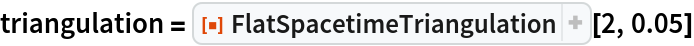Out=Show the causal graph:

 In:=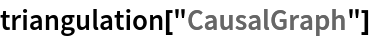Out=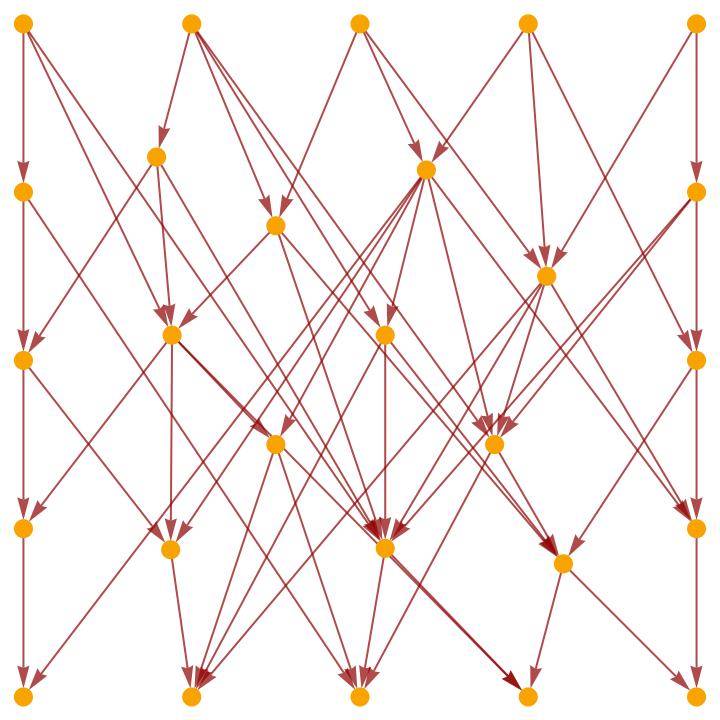Show the full causal graph (without transitive reduction):

 In:=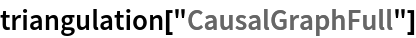Out=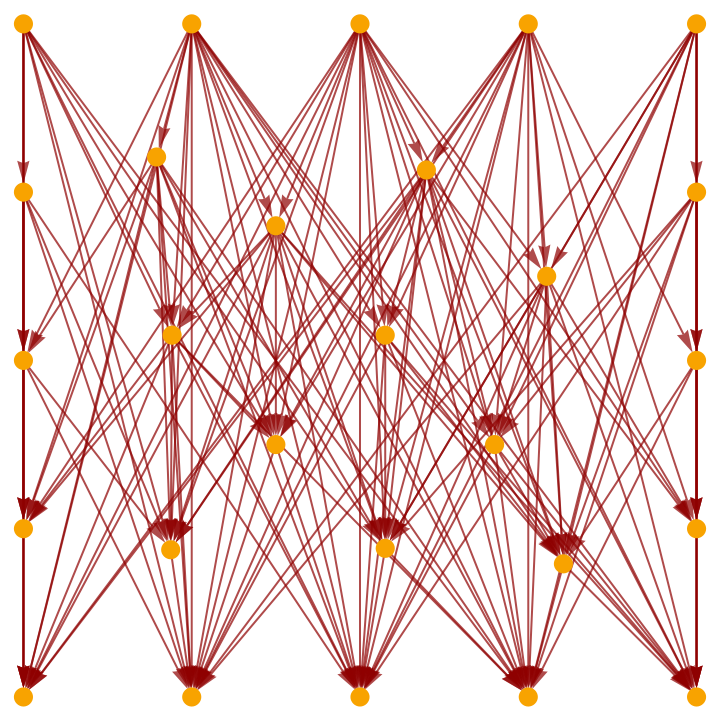Show the causal graph using only triangulation edges:

 In:=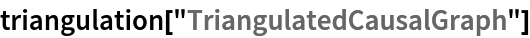Out=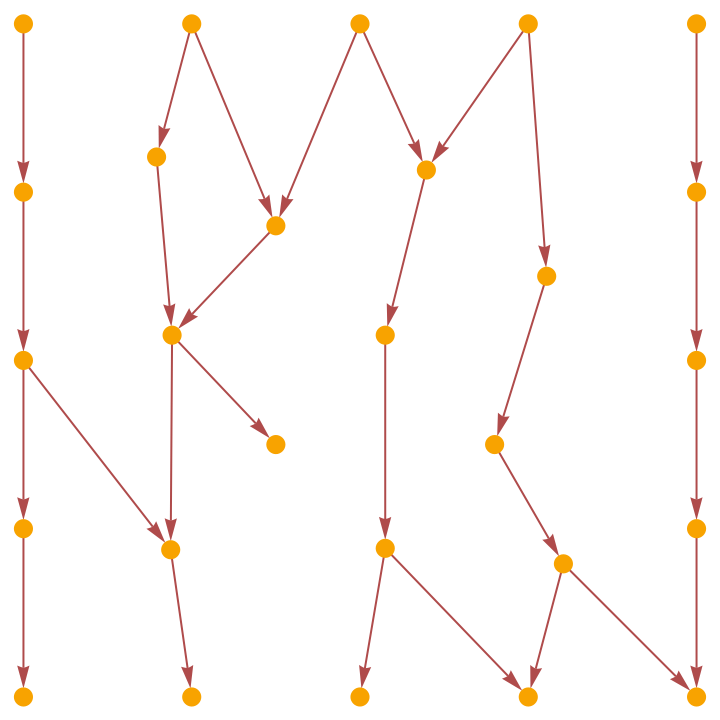Produce a triangulation of a 1+1-dimensional flat (Minkowski) spacetime into smaller simplices of measure (area) up to 0.01 instead:

 In:=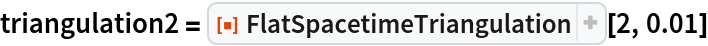Out=Show the causal graph:

 In:=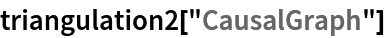Out=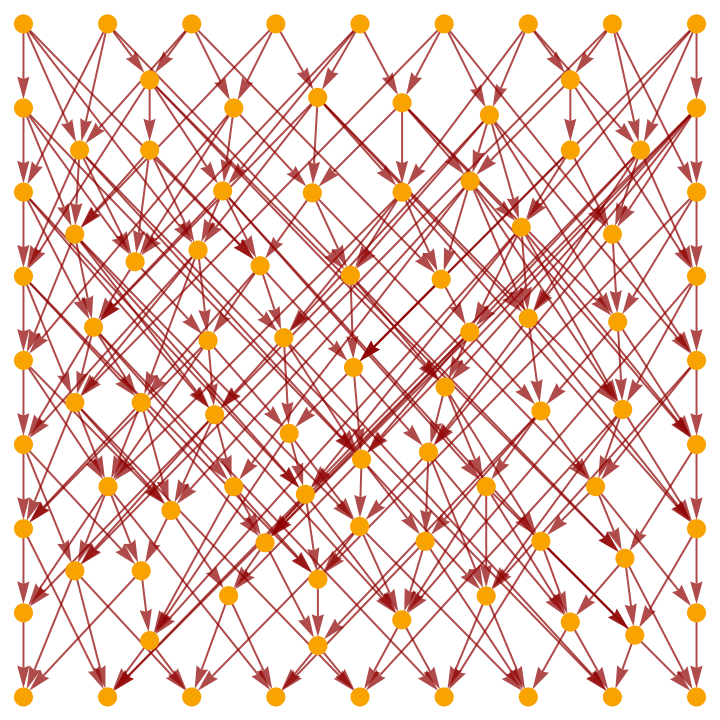Show the mesh region corresponding to the triangulated spacetime surface:

 In:=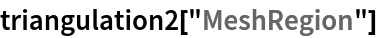Out=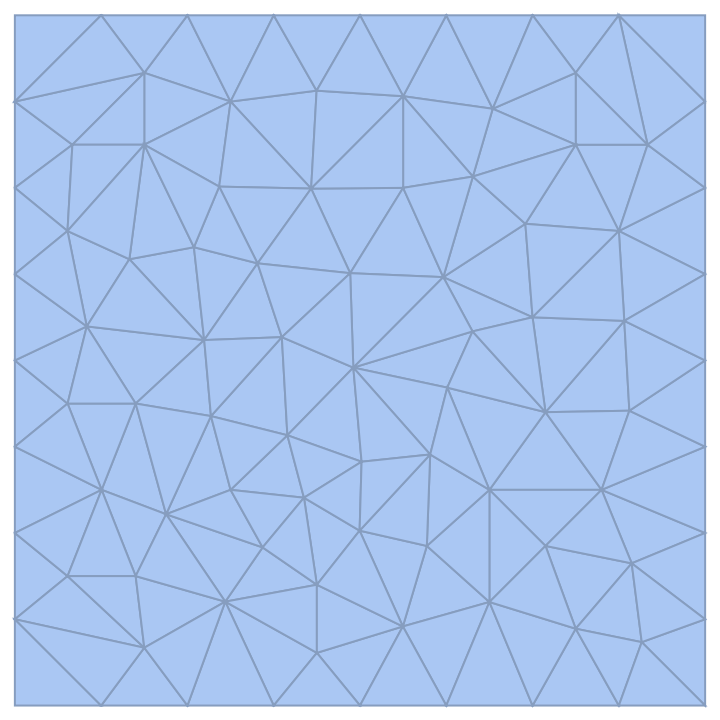Show the connectivity graph of this mesh region:

 In:=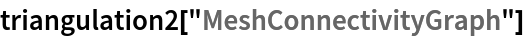Out=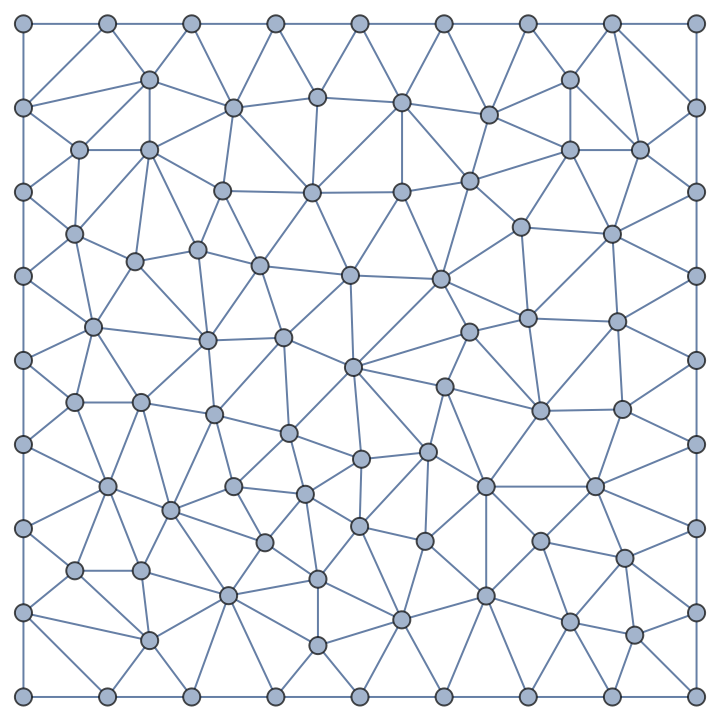Show the full triangulated causal graph (without transitive reduction):

 In:=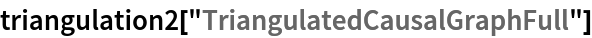Out=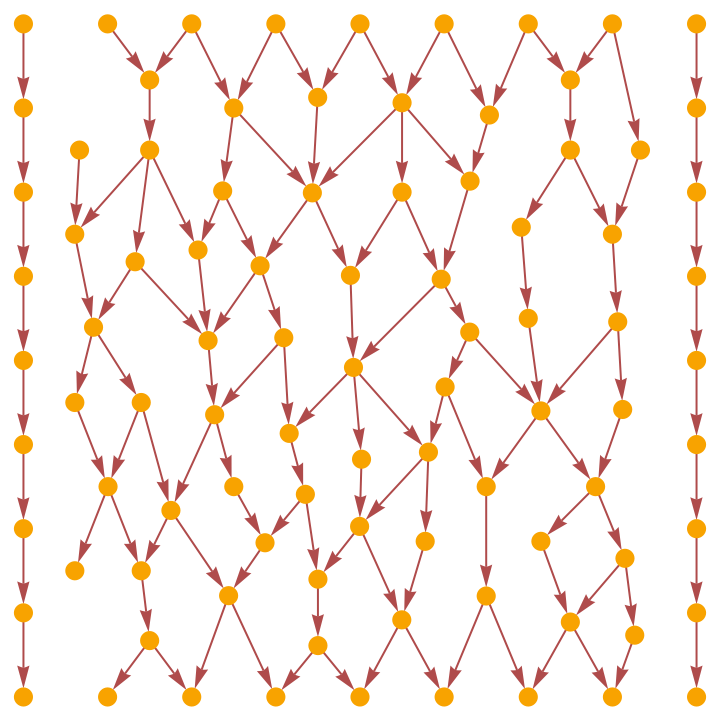Produce a triangulation of a 2+1-dimensional flat (Minkowski) spacetime into simplices of measure (volume) up to 1:

 In:=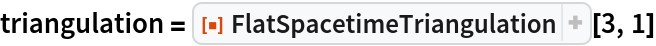Out=Show the causal graph:

 In:=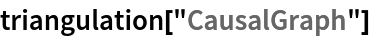Out=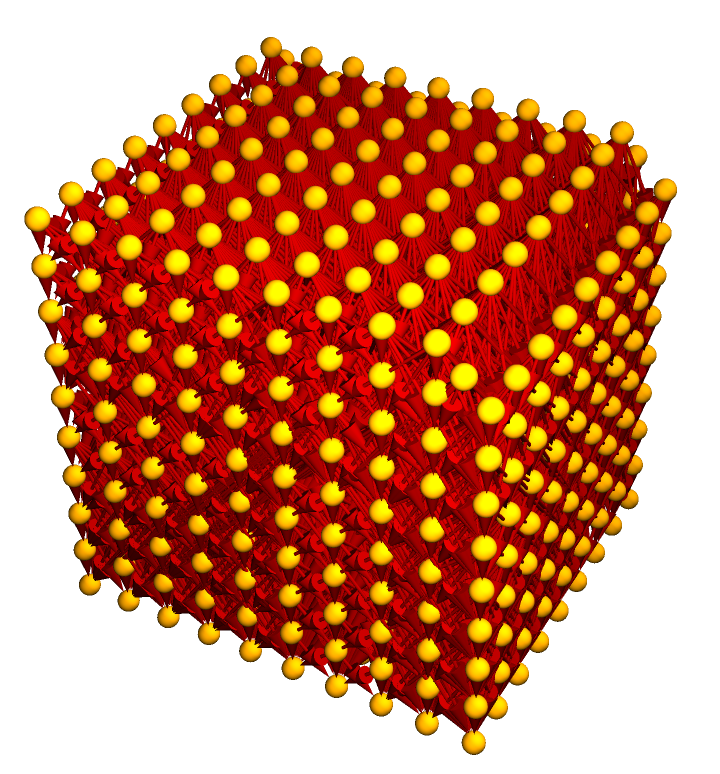Show the pure causal graph (with vertex coordinate information removed):

 In:=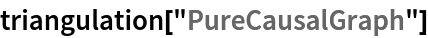Out=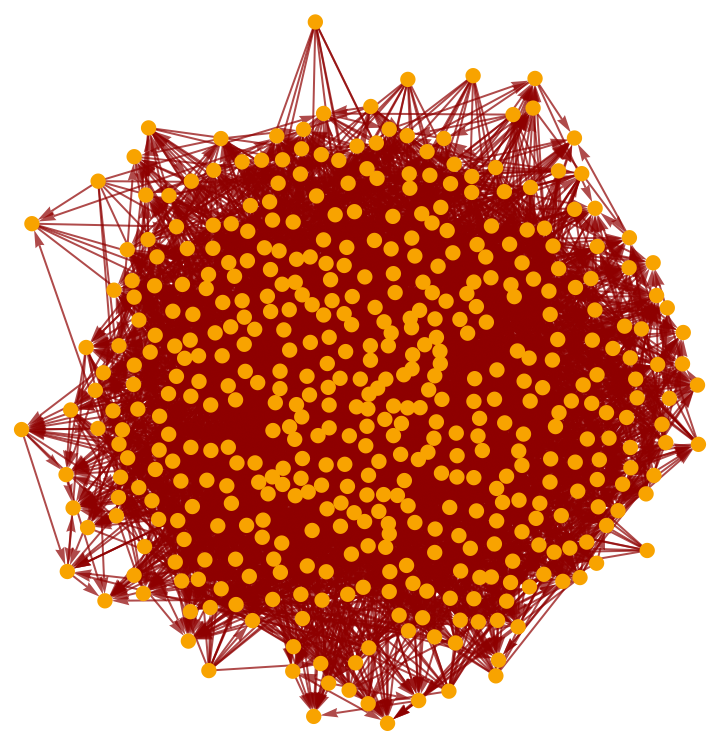Show only the pure triangulated causal graph (with vertex coordinate information removed):

 In:=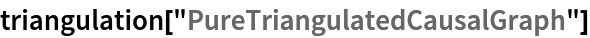Out=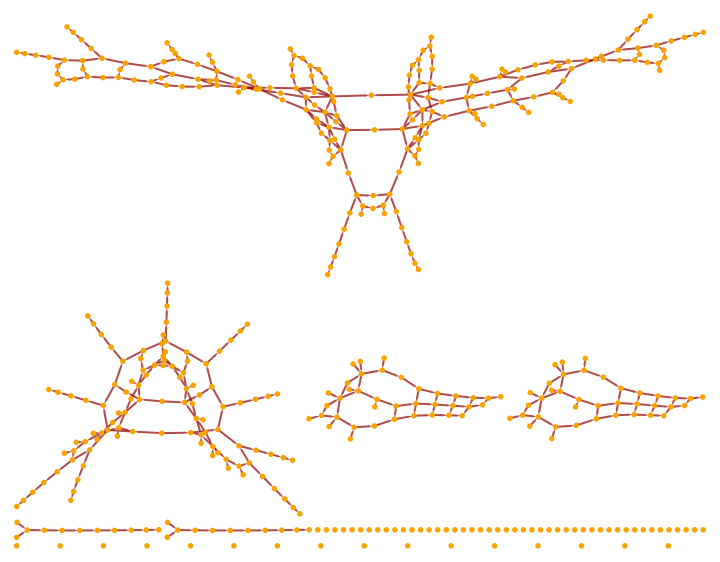### Scope (5)

Triangulations can be produced for 0+1-dimensional spacetimes:

 In:=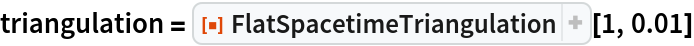Out=Show the pure causal graph:

 In:=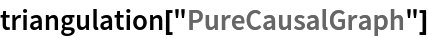Out=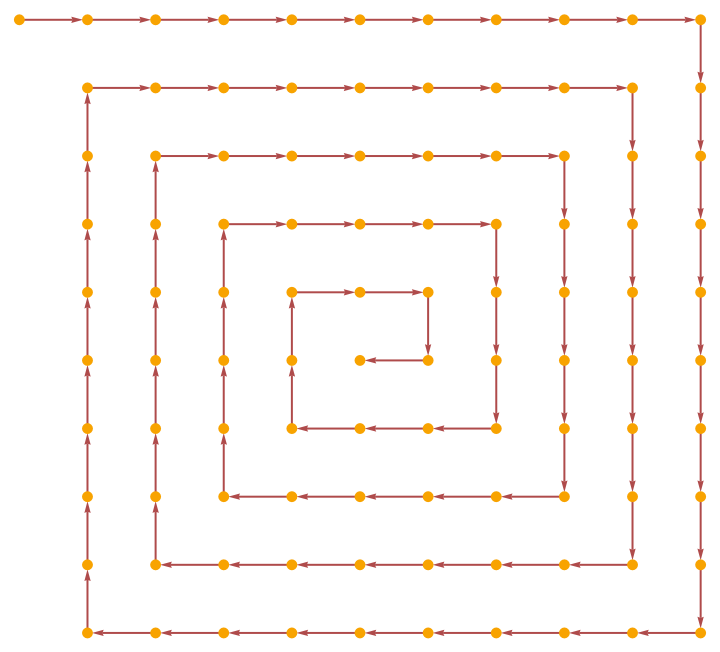Produce a triangulation of a 1+1-dimensional spacetime:

 In:=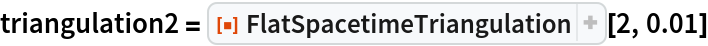Out=Show the pure causal graph:

 In:=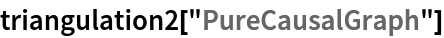Out=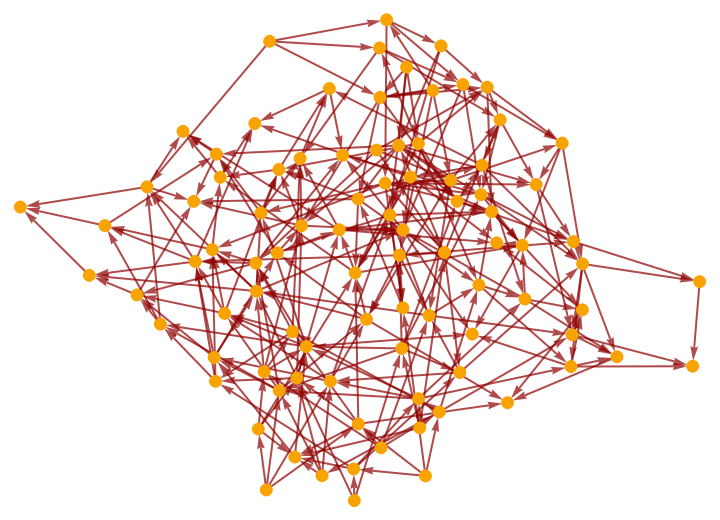Produce a triangulation of a 2+1-dimensional spacetime:

 In:=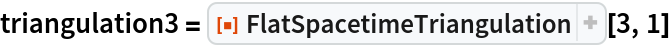Out=Show the pure causal graph:

 In:=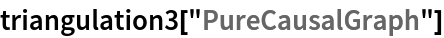Out=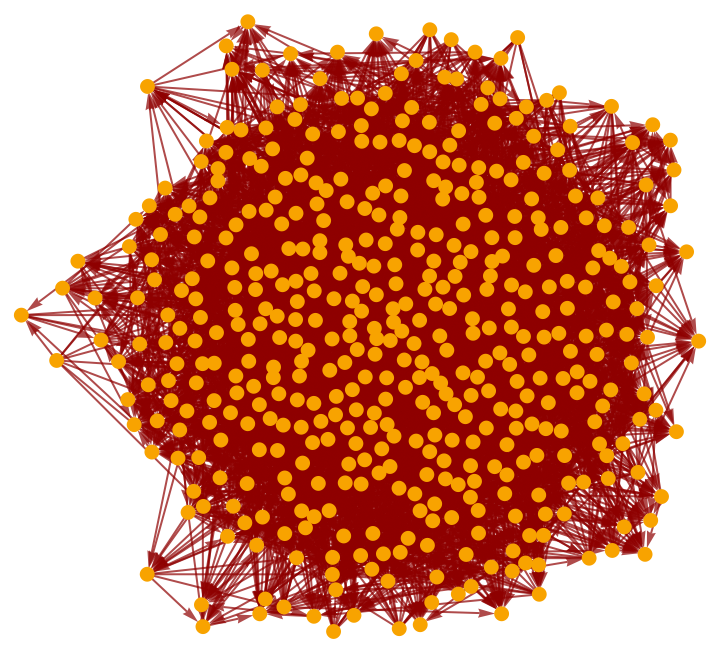Properties can be requested directly from FlatSpacetimeTriangulation:

 In:=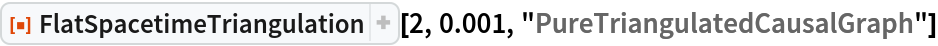Out=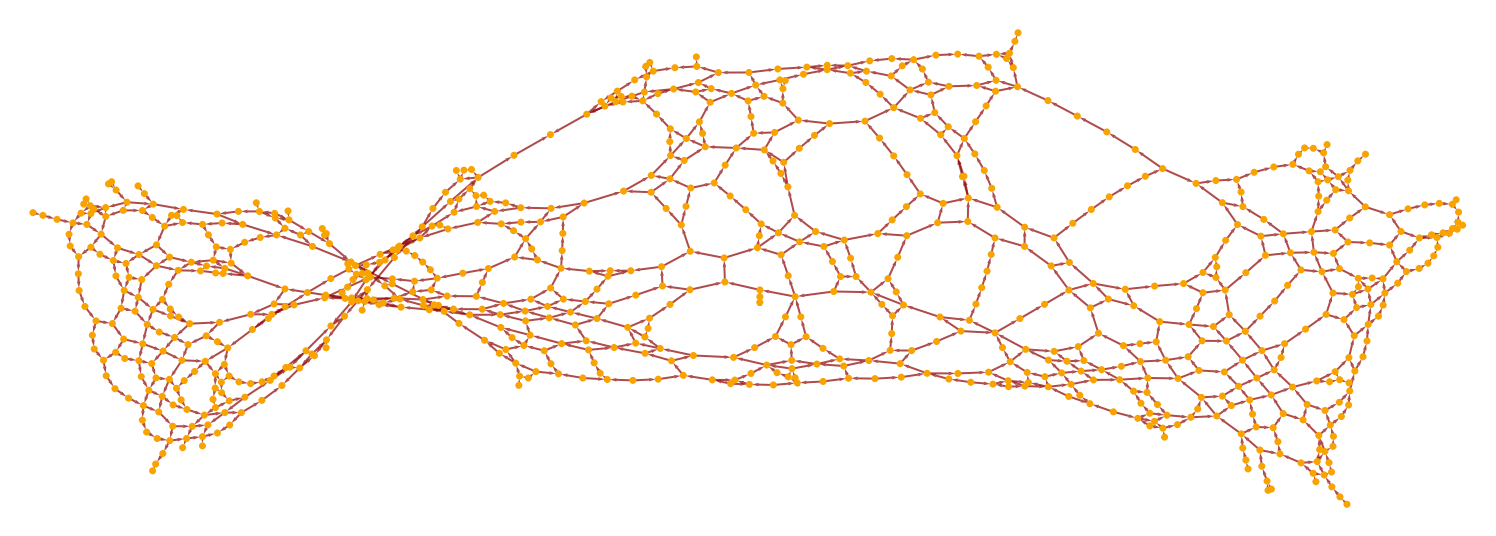Produce a triangulation of a 1+1-dimensional flat (Minkowski) spacetime into simplices of measure (area) up to 0.005:

 In:=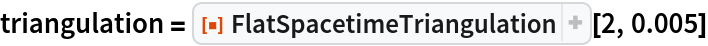Out=Show the complete list of properties:

 In:=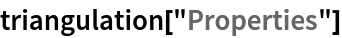Out=Show the full causal graph:

 In:=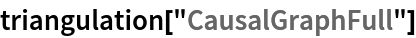Out=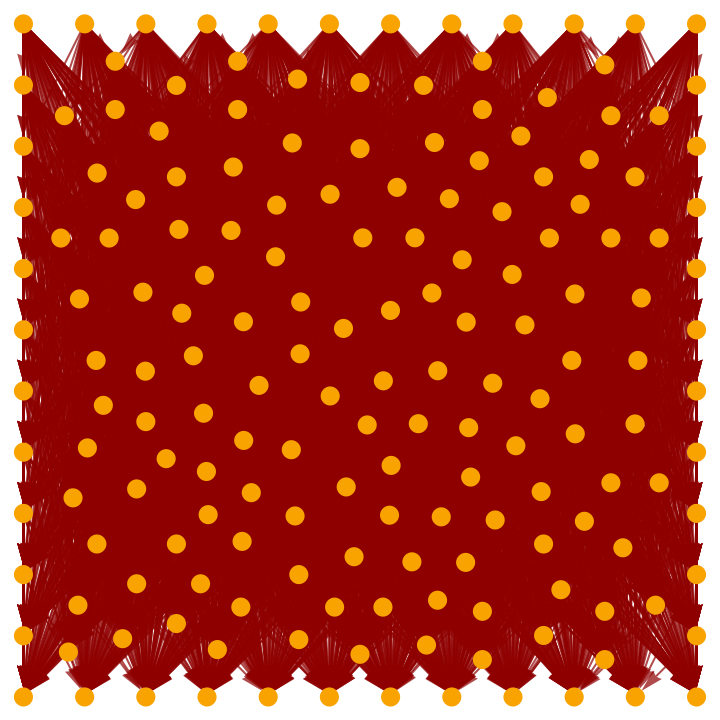Show the transitively-reduced causal graph:

 In:=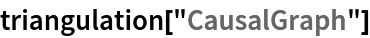Out=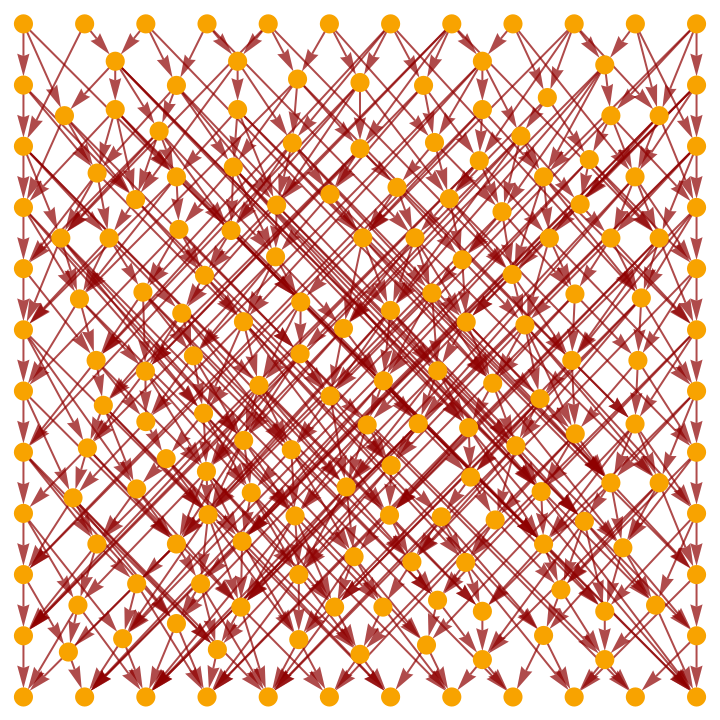Show the full causal graph using only triangulation edges:

 In:=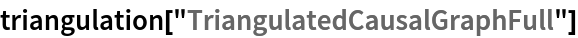Out=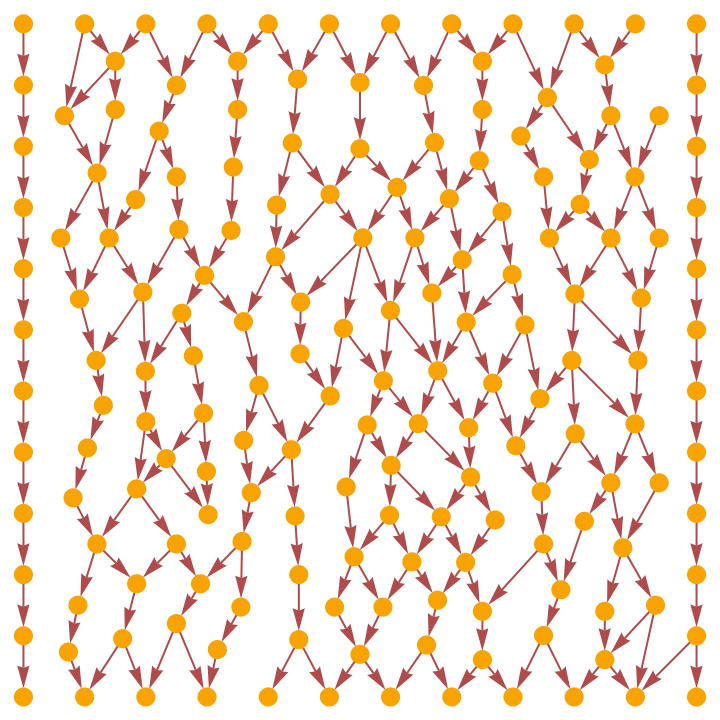Show the transitively-reduced causal graph using only triangulation edges:

 In:=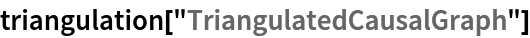Out=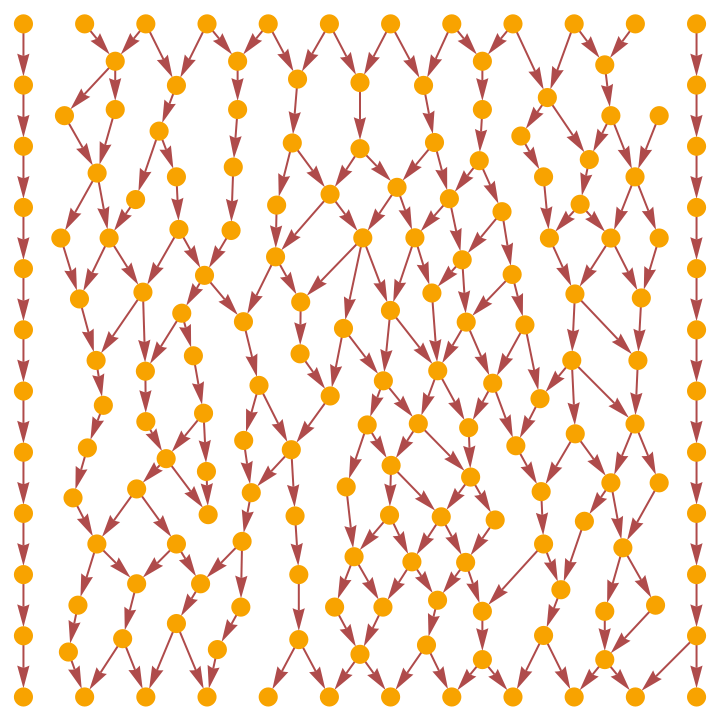Plot the positions of the points in the triangulation only (without causal edges):

 In:=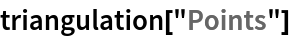Out=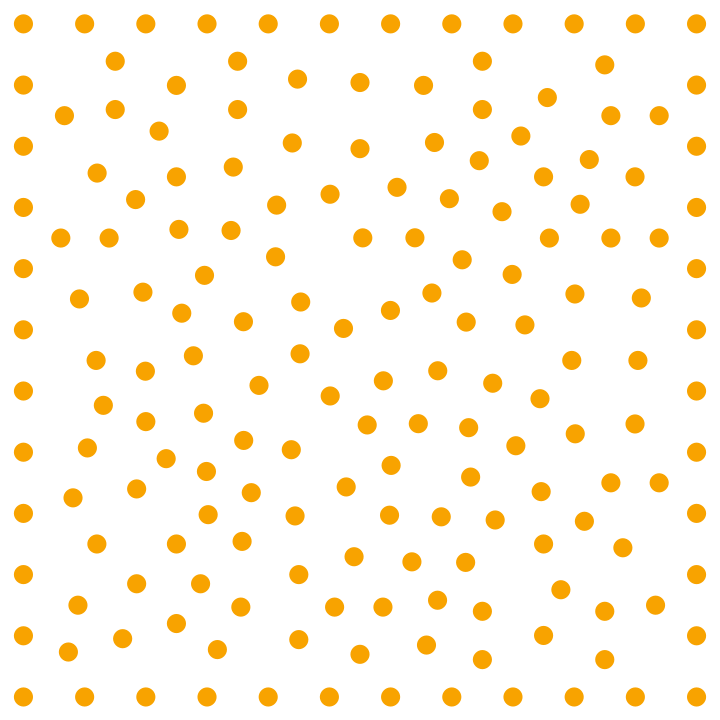Show a complete list of spacetime coordinates for the points in the triangulation (the last coordinate is always the time coordinate):

 In:=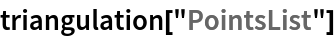Out=Show the number of points in the triangulation:

 In:=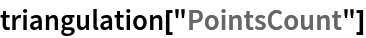Out=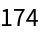Show the number of dimensions in the background spacetime:

 In:=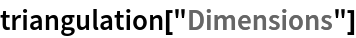Out=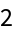Show the first 50 (undirected) edges in the triangulation of the spacetime surface:

 In:=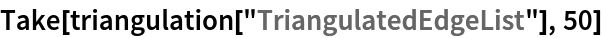Out=Show the total number of (undirected) edges in the triangulation of the spacetime surface:

 In:=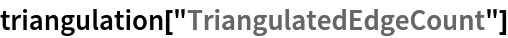Out=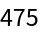Show the maximum measure (in this case, area) of the simplices in the triangulation:

 In:=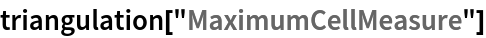Out=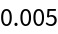Show the total number of causal edges:

 In:=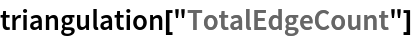Out=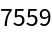Show the number of causal edges after transitive reduction:

 In:=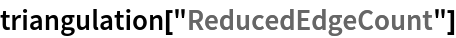Out=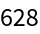Show the total number of causal edges appearing in the triangulation:

 In:=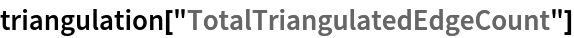Out=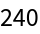Show the number of causal edges appearing in the triangulation after transitive reduction:

 In:=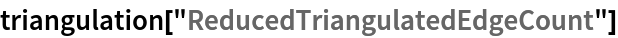Out=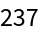Show the pure full causal graph (with vertex coordinate information removed):

 In:=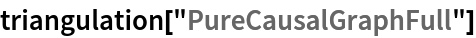Out=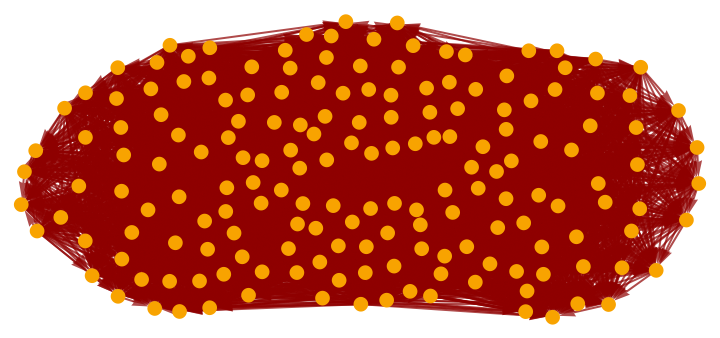Show the pure transitively-reduced causal graph (with vertex coordinate information removed):

 In:=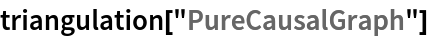Out=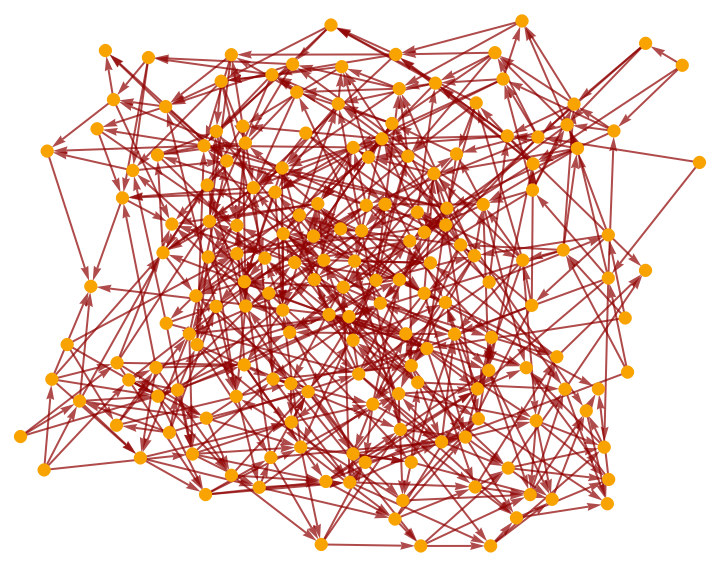Show the pure full causal graph using only triangulation edges (with vertex coordinate information removed):

 In:=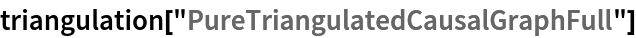Out=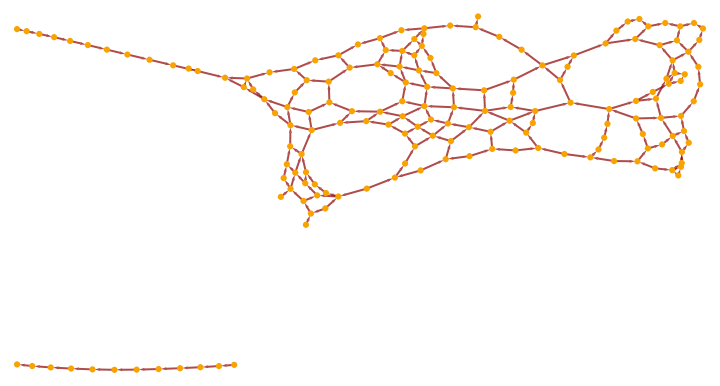Show the pure transitively-reduced causal graph using only triangulation edges (with vertex coordinate information removed):

 In:=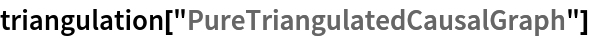Out=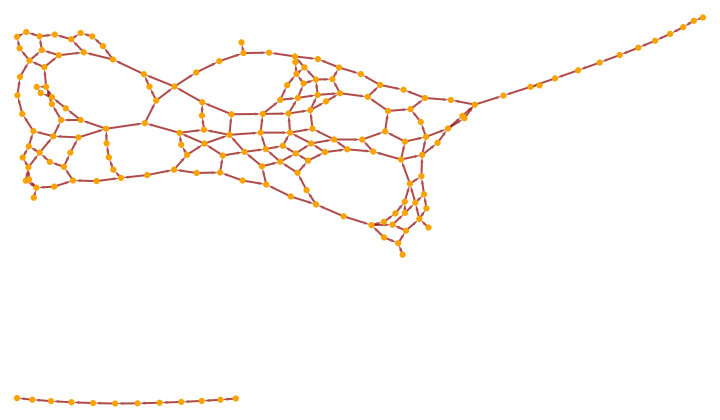Show the mesh region corresponding to the triangulated spacetime surface:

 In:=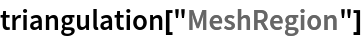Out=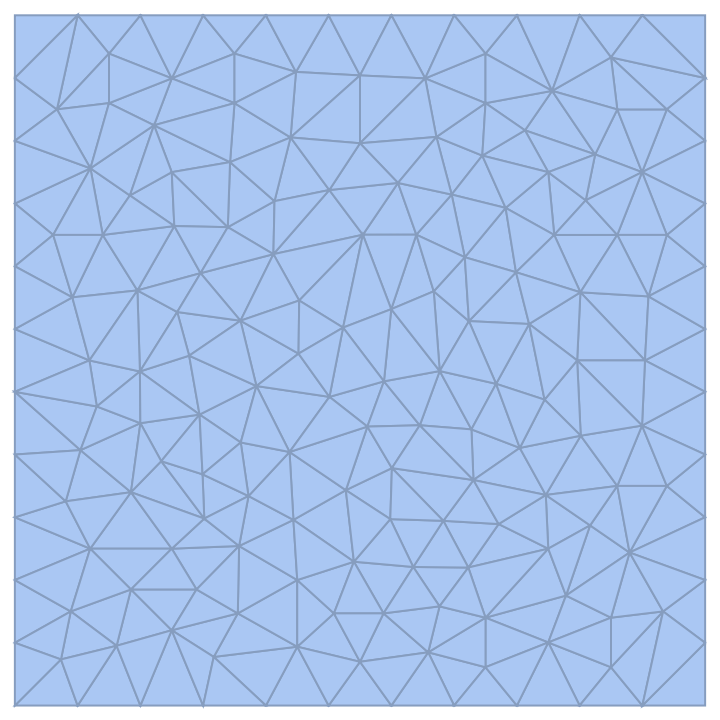Show the connectivity graph of this mesh region:

 In:=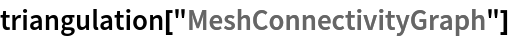Out=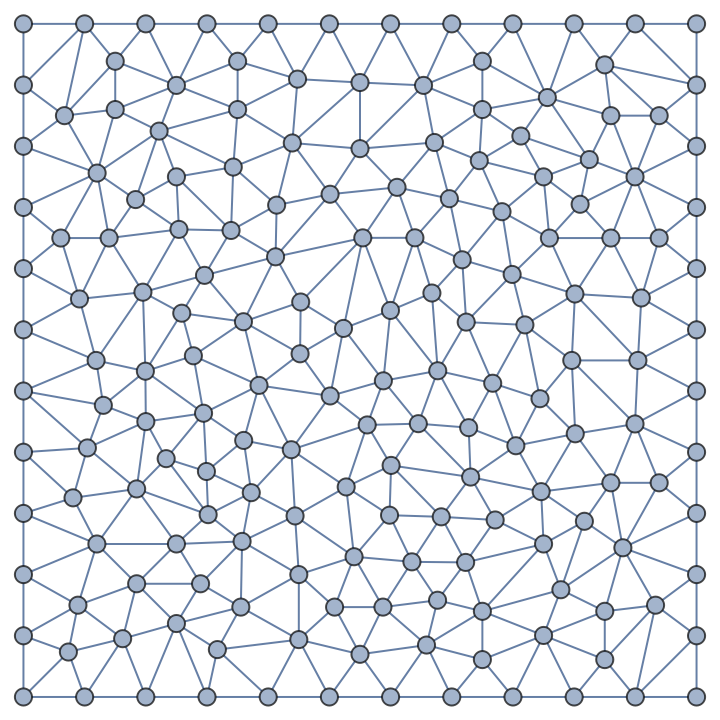Jonathan Gorard

## Version History

• 1.0.0 – 23 July 2021